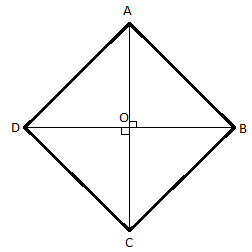QuestionAnswers

# ABCD is a rhombus. Show that diagonal AC bisects ∠A as well as ∠C and diagonal BD bisects ∠B as well as ∠D.Hint: In order to prove that the diagonals AC and BD bisect their respective angles, we use the properties of rhombus, such as all the sides of a rhombus are equal and the diagonals of a rhombus are perpendicular to each other. We also use a property of triangles, which says the corresponding angles of equal sides of a triangle are equal,

Complete step by step solution:
Given Data,
ABCD is a rhombus
AC and BD are the diagonals of the rhombus
With respect to the given data we construct an appropriate figure of rhombus to understand the problem better and solve it accordingly.
Let us consider a rhombus ABCD with diagonals as AC and BD intersecting at the center of the rhombus O.
It looks as follows:According to the property of a rhombus, all the sides of the rhombus are equal, i.e.
AB = BC = CD = DA
Also, the diagonals of a rhombus are not necessarily equal but are perpendicular to each other, i.e.
AC ⊥ BD
From the figure considering the triangle ∆ABC,
AB = BC (∵sides of the rhombus)
∠CAB = ∠ACB (∵corresponding angles of equal sides of triangle are also equal)
AO = OC (∵AC is the diagonal and O is the midpoint of the diagonal)
∠ABO = ∠CBO (∵corresponding angles of equal sides of triangle are also equal)

From the figure considering the triangle ∆ADC,
AD = DC (∵sides of the rhombus)
∠DAC = ∠DCA (∵corresponding angles of equal sides of triangle are also equal)
AO = OC (∵AC is the diagonal and O is the midpoint of the diagonal)
∠CDO = ∠ADO (∵corresponding angles of equal sides of triangle are also equal)

Hence the diagonal AC bisects ∠A as well as ∠C and diagonal BD bisects ∠B as well as ∠D.

Note: In order to solve this type of problems the key is to know the concept and properties of a rhombus and a triangle and also their respective formulae if needed.
We basically use the concept of equal sides of a rhombus and perpendicular diagonals, along with equal sides of triangles corresponding equal angles to prove that each angle divided by the diagonals respectively is exactly divided into two halves.
Each of the angles of the rhombus is 90°, all the angles of a rhombus are equal as well.
View Notes
The Difference Between an Animal that is A Regulator and One that is A ConformerArea of a Rhombus FormulaWhere There is a Will There is a Way EssayDiagonal FormulaLaughter is a Best Medicine EssayDiagonal of Parallelogram FormulaTransistor as a DeviceTypes of AC MotorsPhasor Representation AcCBSE Class 10 Maths Chapter 10 - Circles FormulaImportant Questions for CBSE Class 6 English A Pact with The Sun Chapter 10 - A Strange Wrestling MatchCBSE Class 7 Science Water: A Precious Resource WorksheetsImportant Questions for CBSE Class 6 English A Pact with The Sun Chapter 8 - A Pact with the SunImportant Questions for CBSE Class 10 Maths Chapter 10 - CirclesImportant Questions for CBSE Class 6 English A Pact with The Sun Chapter 1 - A Tale of Two BirdsImportant Questions for CBSE Class 11 Physics Chapter 4 - Motion in a PlaneImportant Questions for CBSE Class 6 English A Pact with The SunImportant Questions for CBSE Class 6 English Honeysuckle Chapter 8 - A Game of ChanceImportant Questions for CBSE Class 11 Business Studies Chapter 7 - Formation of a CompanyImportant Questions for CBSE Class 10 Maths & Science with AnswersCBSE Class 10 Hindi A Question Paper 2020Hindi A Class 10 CBSE Question Paper 2009Hindi A Class 10 CBSE Question Paper 2015Hindi A Class 10 CBSE Question Paper 2016Hindi A Class 10 CBSE Question Paper 2012Hindi A Class 10 CBSE Question Paper 2010Hindi A Class 10 CBSE Question Paper 2007Hindi A Class 10 CBSE Question Paper 2013Hindi A Class 10 CBSE Question Paper 2008Hindi A Class 10 CBSE Question Paper 2014NCERT Solutions for Class 9 English Moments Chapter 8 - A House is Not a HomeRD Sharma Class 12 Maths Solutions Chapter 13 - Derivative as a Rate MeasurerNCERT Solutions Class 11 English Woven Words Essay Chapter 5 What is a Good BookRS Aggarwal Class 8 Mathematics Solutions for Chapter-18 Area of a Trapezium and a PolygonNCERT Solutions for Class 10 English Footprints Without Feet Chapter 10 - The Book that Saved the EarthNCERT Solutions for Class 10 English Footprints Without Feet Chapter 4 - A Question of TrustNCERT Solutions for Class 6 English A pact with the Sun Chapter-10NCERT Solutions for Class 12 English Kaliedoscope Poetry Chapter 4 Kubla Khan or a Vision in a Dream a FragmentNCERT Solutions for Class 6 English Honeysuckle Poem - A House, A HomeNCERT Solutions for Class 10 English First Flight Chapter 1 - A Letter to God